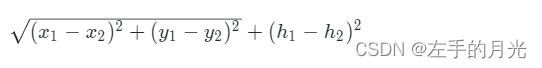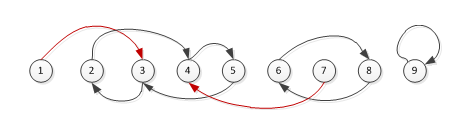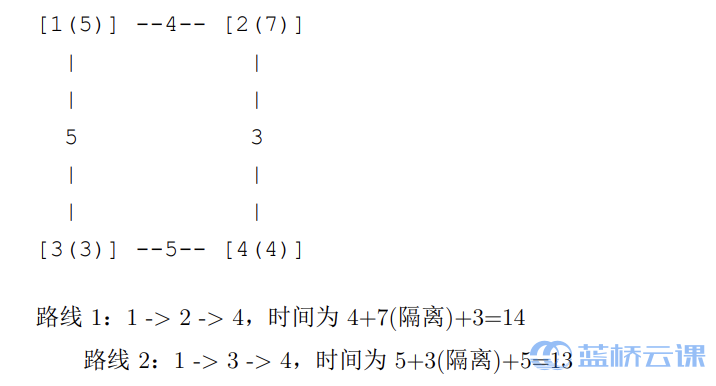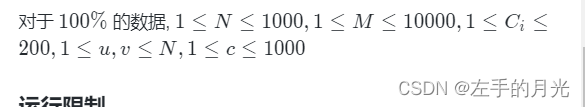## 带刷，带刷，刷起来！！！

2023/9/30 18:02:21

A:::::::::::::::::::通电（最小生成树，Prim，Kruskal）

### 题目描述

2015 年，全中国实现了户户通电。作为一名电力建设者，小明正在帮助一带一路上的国家通电。### 输入输出样例

``````4
1 1 3
9 9 7
8 8 6
4 5 4
``````

``17.41``

``````#include <iostream>
#include <cmath>
#include <vector>
#include <algorithm>
using namespace std;
int n;
struct node{
int x,y,h;
};
struct node1{
int qi,zhong;
double juli;
node1(int qiqi,int zhongzhong,double julijuli){
qi=qiqi;
zhong=zhongzhong;
juli=julijuli;
}
};
int fa;
int find(int x){
if(fa[x]==x) return x;
return fa[x]=find(fa[x]);
}
bool panduan(int x,int y){
int tx=find(x);
int ty=find(y);
if(tx==ty) return false;
fa[tx]=ty;
return true;
}
node dian;
vector<node1> f;
bool cmp(node1 x,node1 y){
return x.juli<y.juli;
}
int main(){
cin>>n;
for(int i=1;i<=n;i++){
int x,y,h;
cin>>x>>y>>h;
dian[i].x=x;
dian[i].y=y;
dian[i].h=h;
}

for(int i=1;i<=n;i++){
for(int j=i+1;j<=n;j++){
double s= sqrt((dian[i].x-dian[j].x)*(dian[i].x-dian[j].x)+(dian[i].y-dian[j].y)*(dian[i].y-dian[j].y))+(dian[i].h-dian[j].h)*(dian[i].h-dian[j].h);
f.push_back(node1(i,j,s));
}
}
sort(f.begin(),f.end(),cmp);
for(int i=1;i<=n;i++){
fa[i]=i;
}
double ans=0;
int len=f.size();
for(int i=0;i<len;i++){
if(panduan(f[i].qi,f[i].zhong)){
ans+=f[i].juli;

}

}
printf("%0.2f",ans);
return 0;
}

``````

### 题目描述

``````#include <iostream>
using namespace std;
int a;
long long ans=1;
int main(){

for(int i=2;i<=100;i++){
int n=i;
for(int j=2;j<=n;j++){
while(n%j==0 && n!=0){
a[j]++;
n=n/j;
}

}
}
for(int i=1;i<=100;i++){
ans=(a[i]+1)*ans;
}
cout<<ans;
return 0;
}``````

C:::::::::::::::::::迷宫（BFS）

### 题目描述

``````010000
000100
001001
110000
``````

``````01010101001011001001010110010110100100001000101010
00001000100000101010010000100000001001100110100101
01111011010010001000001101001011100011000000010000
01000000001010100011010000101000001010101011001011
00011111000000101000010010100010100000101100000000
11001000110101000010101100011010011010101011110111
00011011010101001001001010000001000101001110000000
10100000101000100110101010111110011000010000111010
00111000001010100001100010000001000101001100001001
11000110100001110010001001010101010101010001101000
00010000100100000101001010101110100010101010000101
11100100101001001000010000010101010100100100010100
00000010000000101011001111010001100000101010100011
10101010011100001000011000010110011110110100001000
10101010100001101010100101000010100000111011101001
10000000101100010000101100101101001011100000000100
10101001000000010100100001000100000100011110101001
00101001010101101001010100011010101101110000110101
11001010000100001100000010100101000001000111000010
00001000110000110101101000000100101001001000011101
10100101000101000000001110110010110101101010100001
00101000010000110101010000100010001001000100010101
10100001000110010001000010101001010101011111010010
00000100101000000110010100101001000001000000000010
11010000001001110111001001000011101001011011101000
00000110100010001000100000001000011101000000110011
10101000101000100010001111100010101001010000001000
10000010100101001010110000000100101010001011101000
00111100001000010000000110111000000001000000001011
10000001100111010111010001000110111010101101111000``````

``````#include <iostream>
#include <queue>
using namespace std;
char s={'D','L','R','U'};
int b={{1,0},{0,-1},{0,1},{-1,0}};

struct node{
int x;
int y;
string a;
int bushu;
node(int xx,int yy,string aa,int aaa){
x=xx;
y=yy;
a=aa;
bushu=aaa;
}
};
char a;
bool c;
queue<node> q;
int guding;
void bfs(int xxx,int yyy){
c[xxx][yyy]=1;
q.push(node(xxx,yyy,"",0));
while(!q.empty()){
int xx=q.front().x;
int yy=q.front().y;
if(xx==29 && yy==49){
cout<<q.front().a<<endl;
break;
}
for(int i=0;i<4;i++){
int tx=xx+b[i];
int ty=yy+b[i];
if(tx>=0 && tx<30 && ty>=0 && ty<50 && !c[tx][ty] && a[tx][ty]=='0'){

q.push(node(tx,ty,q.front().a+s[i],q.front().bushu++));
c[tx][ty]=1;
}
}
q.pop();
}
}
int main(){
for(int i=0;i<30;i++){
for(int j=0;j<50;j++){
cin>>a[i][j];
}
}
bfs(0,0);           //(0,0)开始，
return 0;
}``````

D:::::::::::::::::::发现环（并查集，DFS）

### 输入输出样例

``````5
1 2
3 1
2 4
2 5
5 3
``````

``1 2 3 5``

``````#include <iostream>
#include <vector>
#include <algorithm>
#include <cstring>
using namespace std;
int n;
int fa;
bool vis;
int find(int x){
if(fa[x]==-1) return x;
return fa[x]=find(fa[x]);
}
vector<int> s;

int qi,end1;
int flage;
bool pl;
int j;
int mm=0;

void print(int step)
{
sort(j+1,j+1+step);
for(int i=1;i<=step;i++)
cout<<j[i]<<" ";
}

void dfs(int x,int step)
{
vis[x]=1;
j[step]=x;
if(x==end1){
print(step);
return;
}
for(int i=0;i<s[x].size();i++)
if(!vis[s[x][i]]){
dfs(s[x][i],step+1);
}
}

int main(){
cin>>n;
memset(fa,-1,sizeof(fa));
for(int i=0;i<n;i++){
int a,b;
cin>>a>>b;

if(find(a)==find(b)){
qi=a,end1=b;
}else{
fa[find(a)]=b;
s[a].push_back(b);
s[b].push_back(a);
}
}
dfs(qi,1);
return 0;
}
``````

E:::::::::::::::::::大臣的旅费（双DFS）

### 题目描述

J 是 T 国重要大臣，他巡查于各大城市之间，体察民情。所以，从一个城市马不停蹄地到另一个城市成了 J 最常做的事情。他有一个钱袋，用于存放往来城市间的路费。

J 大臣想知道：他从某一个城市出发，中间不休息，到达另一个城市，所有可能花费的路费中最多是多少呢？

### 输入输出样例

``````5
1 2 2
1 3 1
2 4 5
2 5 4
``````

``````135
``````

这道题好恶心，用dfs最后一个例题，数值较大，开足内存卡内存，不开内存，运行错误，用两遍dfs。

``````#include <iostream>
#include <vector>
#include <algorithm>
#include <cstring>
using namespace std;
int n;
int a;
vector<int> g;
int c;

bool vis;
long long ans;
long long p;
void dfs(int qi,int zhong,long long bu){
if(bu>ans){
return;
}
if(qi==zhong){
ans=min(ans,bu);

p=max(p,ans);
return;
}
int len=g[qi].size();
for(int i=0;i<len;i++){
if(!vis[g[qi][i]]){
vis[g[qi][i]]=1;
dfs(g[qi][i],zhong,bu+a[qi][g[qi][i]]);
vis[g[qi][i]]=0;
}
}
}
int main(){
cin>>n;
if(n==1 || n==0){
cout<<0;
return 0;
}
for(int i=1;i<n;i++){
int p,q,d;
cin>>p>>q>>d;
a[p][q]=a[q][p]=d;
g[p].push_back(q);
g[q].push_back(p);
}

for(int i=1;i<=n;i++){
for(int j=i+1;j<=n;j++){
ans=1e9;
vis[i]=1;
dfs(i,j,0);

vis[i]=0;
c++;
}
}

cout<<p*10+p*(p+1ll)/2;

return 0;
} ``````

``````
#include <iostream>
#include<vector>
using namespace std;
const int N =100010;

int n;
struct Edge
{
int id,w;
};
vector<Edge> h[N];
int dist[N];

void dfs(int u,int father,int distance)
{
dist[u] = distance;

for(auto node : h[u])
if(node.id!= father)
dfs(node.id,u,distance + node.w);
}

int main()
{
scanf("%d",&n);
for(int i=0;i<n-1;i++)
{
int a,b,c;
scanf("%d%d%d",&a,&b,&c);
h[a].push_back({b,c});
h[b].push_back({a,c});

}

dfs(1,-1,0);

int u=1;
for(int i=1;i<=n;i++)
if(dist[i]>dist[u]) u=i;

dfs(u,-1,0);

for(int i=1;i<=n;i++)
if(dist[i]>dist[u]) u=i;
int v = dist[u];

printf("%lld\n",10*v + v*(v+1ll)/2);
return 0;
}``````

F:::::::::::::::::::最小公倍数（高精度）

### 题目描述

69720375229712477164533808935312303556800

### 输入输出样例

``````6
``````

``60``
`````` #include<iostream>
#include<cstring>
using namespace std;
int prime,ans=0;
void prime_it(){
for(int i=1;i<=100;i++)prime[i]=i;
for(int i=2;i<=100;i++){
for(int j=2;j<=i-1;j++)if(prime[i]%prime[j]==0)prime[i]/=prime[j];
}
}
int num,len=1;
int main(){
int n;
prime_it();
while(cin>>n){
memset(num,0,sizeof(num));
num=1,len=1;
for(int i=1;i<=n;i++){
for(int j=1;j<=len;j++){
num[j]*=prime[i];
}
for(int j=1;j<=len;j++){
num[j+1]+=num[j]/10;
num[j]%=10;
}
while(num[len+1]){
len++;
num[len+1]=num[len]/10;
num[len]%=10;
}
}
for(int i=len;i>=1;i--)cout<<num[i];
cout<<endl;
}
return 0;
} ``````

G:::::::::::::::::::排列叙述（全排列）

abcd 0

abdc 1

acbd 2

acdb 3

bacd 6

bcda 9

bdac 10

bdca 11

cabd 12

cbda 15

cdab 16

cdba 17

### 输入输出样例

``````bdca
``````

``11``

``````#include <iostream>
#include <string>
#include <algorithm>
using namespace std;
string arr;
long long ans=0;
int main(){
cin>>arr;
string arr1=arr;
sort(arr1.begin(),arr1.end());
if(arr1==arr){
cout<<0;
return 0;
}
while(next_permutation(arr1.begin(),arr1.end())){
if(arr1==arr){
break;
}
ans++;
}
cout<<ans+1;
return 0;
}``````

H:::::::::::::::::::小朋友崇拜圈（DFS）

### 输入输出样例

``````9
3 4 2 5 3 8 4 6 9
``````

``````4
``````### 运行限制

• 最大运行时间：1s
• 最大运行内存: 256M
``````#include <iostream>
using namespace std;
int a;
bool vis;
int n;
bool p;
int ans;
void dfs(int x,int y,int an){
if(x==y && an>1){
ans=max(ans,an);
return;
}
if(!vis[x]){
vis[x]=1;
dfs(a[x],y,an+1);
vis[x]=0;
}

}
int main(){
cin>>n;
for(int i=1;i<=n;i++){
cin>>a[i];
}
for(int i=1;i<=n;i++){
p=false;
dfs(i,i,0);
}
cout<<ans;
return 0;
}
``````

I:::::::::::::::::::出差（Dijkstra

### 问题描述

A 国有 N 个城市, 编号为 1…N 。小明是编号为 1 的城市中一家公司的员 工, 今天突然接到了上级通知需要去编号为 N 的城市出差。

### 样例输入

``````4 4
5 7 3 4
1 2 4
1 3 5
2 4 3
3 4 5
``````

### 样例输出

``````13
``````

### 样例说明### 评测用例规模与约定### 运行限制

• 最大运行时间：1s
• 最大运行内存: 512M
``````#include <iostream>
#include <cstring>
#include <cmath>
using namespace std;
int n,m;     //n个城市，m条路线
int geli;   //到每个城市的隔离时间
int luxian;
int juli;          //到一点的最短距离
bool vis;
int dj(){
memset(juli,0x3f3f3f3f,sizeof(juli));
juli=0;      //距离起点为0
geli=0;      //出自己城市不隔离
geli[n]=0;      //到达终点不需要隔离
for(int i=1;i<=n;i++){
int s=-1;
for(int j=1;j<=n;j++){
if(!vis[j]&&((s==-1)||(juli[j]<juli[s]))){
s=j;
}
}
vis[s]=1;
for(int j=1;j<=n;j++){

juli[j]=min(juli[j],juli[s]+luxian[s][j]+geli[s]);

}
}
return juli[n];
}
int main(){
cin>>n>>m;
for(int i=1;i<=n;i++){
cin>>geli[i];
}
memset(luxian,0x3f3f3f3f,sizeof(luxian));  //无穷大
for(int i=1;i<=n;i++){
luxian[i][i]=0;                    //自己到自己为0
}
for(int i=0;i<m;i++){
int a,b,c;
cin>>a>>b>>c;
luxian[a][b]=luxian[b][a]=min(c,luxian[a][b]);
}
int ans=dj();
cout<<ans;
return 0;
}``````

J:::::::::::::::::::百亿富翁（单调栈）

### 输入描述

1≤N≤7×105，1≤hi​≤109。

### 输入输出样例

``````5
3 1 2 5 4
``````

``````-1 1 1 -1 4
4 3 4 -1 -1``````

``````#include <iostream>
#include <stack>
using namespace std;
int n;
long long a;
stack<int> st;
int l;
int r;
int main(){
cin>>n;
for(int i=1;i<=n;i++){
cin>>a[i];
}
for(int i=1;i<=n;i++){
while(st.size() && a[st.top()]<=a[i]) st.pop();
if(st.size()){
l[i]=st.top();
}else{

l[i]=-1;
}
st.push(i);
}

while(!st.empty()){
st.pop();
}

for(int i=n;i>=1;i--){
while(st.size() && a[st.top()]<=a[i]) st.pop();
if(st.size()){
r[i]=st.top();
}else{
r[i]=-1;
}
st.push(i);
}
for(int i=1;i<=n;i++){
cout<<l[i]<<' ';
}
cout<<endl;
for(int i=1;i<=n;i++){
cout<<r[i]<<' ';
}
return 0;
}
``````

### C的实用笔记37——几种常用的字符串处理API（二）

6.字符串拼接函数 0、知识点&#xff1a; 内存污染&#xff08;存储字符串的目的内存不够用时&#xff0c;后面的内存会被污染&#xff0c;也就是被修改&#xff0c;类似于下标越界&#xff09; strcat其实就是另一种形式的strcpy&#xff0c;一个从末尾开始复制&#xff0c;一…

### [综]Review of bike-sharing system studies using bibliometrics method

Review of bike-sharing system studies using bibliometrics method 文献计量学方法在自行车共享系统研究中的应用 article{2022reviewbikesharing, title {Review of bike-sharing system studies using bibliometrics method}, journal {Journal of Traffic and Transport…

### (Cont.) OpenPose OpenCV Caffe QT

Cont. conda deactivate Remember to deactivate conda before compiling conda deactivatecaffe colas.h Path: openpose/3rdparty/caffe/include/cblas.h Case 1: 如果没有 寻找一下 &#xff08;大概率在python directory有&#xff09;复制过来即可 exactly寻找命令&am…

### 用户体验设计中的颜色

&#x1f525;1 颜色模型是一种抽象的用于描述颜色被定义和呈现方式的数学模型。RGB模型&#xff08;加色模式&#xff09;最多用于显示器上&#xff0c;因为它是发光的颜色。CMYK模型&#xff08;减色模式&#xff09;适用于印刷体&#xff0c;不会发光而是反光的模式&#x1…

### Linux使用：环境变量指南和CPU和GPU利用情况查看

Linux使用&#xff1a;环境变量指南和CPU和GPU利用情况查看Linux环境变量初始化与对应文件的生效顺序Linux的变量种类设置环境变量直接运行export命令定义变量修改系统环境变量修改用户环境变量修改环境变量配置文件环境配置文件的区别profile、 bashrc、.bash_profile、 .bash…

### 苦中作乐---竞赛刷题（15分-20分题库）

&#xff08;一&#xff09;概述 &#xff08;Ⅰ&#xff09;彩票是幸运的 &#xff08;Ⅱ&#xff09;AI 英文问答程序 &#xff08; Ⅲ &#xff09; 胎压检测 &#xff08;二&#xff09;题目 Ⅰ 彩票的号码有 6 位数字&#xff0c;若一张彩票的前 3 位上的数之和等于后 3 …

### c++多线程 1

https://www.runoob.com/cplusplus/cpp-multithreading.html 两种类型的多任务处理&#xff1a;基于进程和基于线程。 基于进程的多任务处理是程序的并发执行。 基于线程的多任务处理是同一程序的片段的并发执行。 线程 c11以后有了 标准库 1 函数 2 类成员函数 3 lambda函…

### BC12 字符圣诞树

BC12 字符圣诞树 我们先观察一下这个图&#xff0c;拆分来看&#xff0c;就是下面这张图 这么看来就是每行都有9个元素&#xff0c;字符或空格&#xff0c;只是他俩每行的所占比不同&#xff0c; 我们观察一下规律&#xff0c;发现 &#xff08;我们只用观察最后一个字符前面的…

### Power BI----DAX 再复习（DAX基础、基础函数）

DAX基础一、数据模型模型关系二、基础函数1.时间日期函数2.关系函数3. 转换函数4.文本函数5.聚合函数6.逻辑函数7.三角函数和数学函数8.信息函数DAX 全称 Data Analysis eXpressions&#xff0c;是面向 Microsoft Power BI、Microsoft SQL Server Analysis Services (SSAS)和 M…